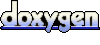# XMath::Vector3 Class Reference [XMath]

`#include <Vector3.h>`

## Detailed Description

Author:
Koray Balci
Version:
1.0
Date:
April 2002
Class that represents a vector in 3D, and necessary mathematical operations.
Note:
A small bit of advice can be usage of *=, +=, -= instead of *, +, - for optimization purposes. Because, each time the latter versions are called, a new Vector3 object is created, and returned.

## Public Member Functions

Vector3operator/= (float scalar)
/= operator overloaded for scalar division
Vector3 operator/ (float scalar) const
/ operator overloaded for scalar division
Vector3 operator- () const
Negation operation overloaded.
Vector3operator-= (const Vector3 &rhs)
-= operator overloaded
Vector3operator+= (const Vector3 &rhs)
+= operator overloaded
Vector3 operator- (const Vector3 &rhs) const
subtraction operator overloaded
Vector3 operator+ (const Vector3 &rhs) const
+ operator overloaded
Vector3normalize (float tolerance=1e-06)
Unitize the current vector.
float lengthSqr () const
Computes square of magnitude of the vector.
float length () const
Computes magnitude of the vector.
Vector3operator *= (const float scalar)
*= operator for scalar multiplication
Vector3 operator * (const float scalar) const
* operator for scalar multiplication
Vector3 unitCross (const Vector3 &rhs) const
Cross Product with a unit vector output.
Vector3 cross (const Vector3 &rhs) const
Cross Product.
float dot (const Vector3 &rhs) const
Dot Product.
bool operator!= (const Vector3 &rhs) const
!= operator overloaded
bool operator== (const Vector3 &rhs) const
== operator overloaded
Vector3operator= (const Vector3 &rhs)
= operator overloaded
Vector3 (const Vector3 &pCopy)
Copy Constructor.
Vector3 (const float ix, const float iy, const float iz)
Additional Constructor.
Vector3 ()
Default Constructor.
~Vector3 ()
Destructor.
operator float * ()
float* type cast operator
operator const float * () const
const float* type cast operator

## Public Attributes

float x
x component in 3D space
float y
y component in 3D space
float z
z component in 3D space

## Member Function Documentation

 Vector3 & XMath::Vector3::normalize ( float tolerance = `1e-06` ) ` [inline]`

Makes the current Vector a unit vector.

Parameters:
 tolerance If the actual magnitude is smaller than this value, unitizing cannot be done, and a value of 0 magnitude is returned.
Returns:
Returns the old magnitude (or 0 if too small before) of the vector

 Vector3 XMath::Vector3::unitCross ( const Vector3 & rhs ) const` [inline]`
 A special version of cross multiplicatio that returns a unit vector as a result. Might be handy for lighting calculations especially. Returns:A unit vector perpendicular to the plane defined by this vector and the vector passed as parameter.

The documentation for this class was generated from the following file:
Generated on Mon Aug 28 15:39:26 2006 for Xface Core Library by1.4.6-NO